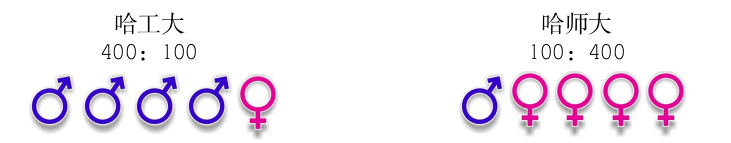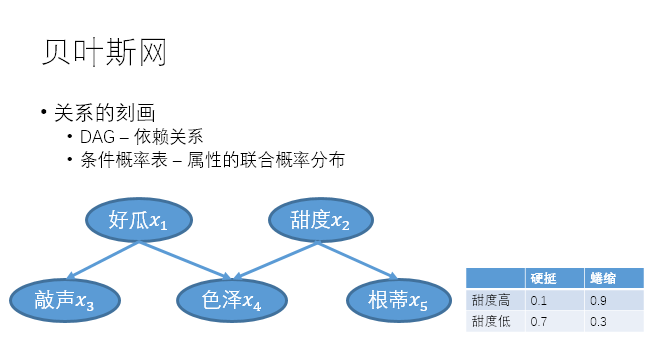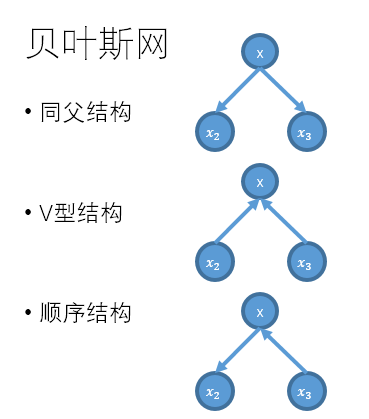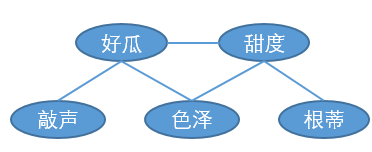# 贝叶斯分类器

1. 贝叶斯决策论
2. 朴素贝叶斯分类器
3. 半朴素贝叶斯分类器
4. 贝叶斯网络

### 1. 贝叶斯决策论

Example

哈工大与哈师大的同学举办大型联♂谊♀会，两个学校分别有500人参与。在联谊会上随机找到一个同学，请猜测他是那个学校的学生？• P(工大学生=是 | 性别 = X)
• P(师大学生=是 | 性别 = X)

$$P(c|\boldsymbol{x})=\frac{P(\boldsymbol{x}, c)}{P(\boldsymbol{x})}=\frac{P(c)P(\boldsymbol{x}|c)}{P(\boldsymbol{x})}$$

### 2. 朴素贝叶斯分类器

$P(c|\boldsymbol{x})=\frac{P(c)}{P(\boldsymbol{x})}\prod_{i=1}^{d}P(x_i|c)$

$p(x_i|c)=\frac{1}{\sqrt{2\pi}\sigma_{c,i}}exp(-\frac{(x_i-\mu_{c,i})^2}{2\sigma^2_{c,i}})$

$P(c)=\frac{|D_c|+1}{|D|+N}$ $P(x_i|c)=\frac{|D_{c,xi}|+1}{|D_c|+N_i}$

### 2. 半朴素贝叶斯分类器

$P(c|x)\propto P(c)\prod_{i=1}^{d}P(x_i|c,pa_i)$

1. 计算每两个属性之间的条件互信息
2. 以属性为节点构建完全图，边的权重为条件互信息
3. 构建完全图的最大生成树，从中选择一个节点为根节点，把所有的边改为有向边，方向为由根节点向外的方向。

TAN只保留了强相关属性之间的依赖性。

Average ODE

AODE采取的方案是去掉出现次数过少的属性，将剩余的属性集成起来，使用公式表达如下：

$P(c|x)\propto \sum_{i=1;|D_{xi}|\geq m}^d P(c,x_i)\prod_{j=1}^{d}P(x_j|c,x_i)$

### 贝叶斯网$B=\langle G,\Theta \rangle$1. 找出有向图中所有的V型结构
2. 将父节点之间添加一条无香边
3. 所有有向边改为无向边##### 学习

$s(B|D)=f(\theta)|B|+LL(B|D)$

##### EM算法

$LL(\Theta|X)=lnP(X|\Theta)=ln\sum_ZP(X,Z|\Theta)$

EM算法分为两步：

• E步：根据上一次计算得到的$$\Theta$$，计算Z的期望。对于首次计算，使用初值$$\Theta^0$$
• M步：根据Z的期望计算$$\Theta$$的最大似然估计